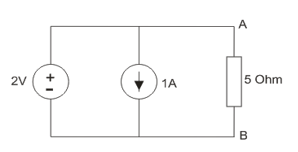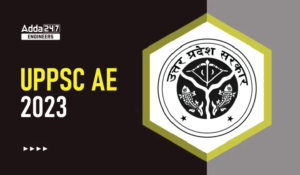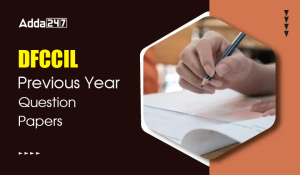Engineering Jobs   »   DFCCIL ELECTRICAL QUIZ,

# DFCCIL’21 EE: Daily Practices Quiz 20-July-2021

Each question carries 1 mark.
Negative marking: 1/4 mark
Total Questions: 06
Time: 08 min.

Q1. “Overshoot” in time response of control systems is an indication of:
(a) Final value of the output
(b) Measure of relative stability
(d) None of the above

Q2. Find the current through 5 Ohm resistors from A to B.(a) -0.4 A
(b) -1.4 A
(c) 0.4 A
(d) 1.4 A

Q3. Determine the deflection factor (in V/m) of a CRO, when the deflection sensitivity of the CRO is 5 m/V.
(a) 0.25
(b) 0.35
(c) 0.20
(d) 0.40

Q4. A 10 Ω resistive load is to be impedance matched by a transformer to a source with 6250 Ω of internal resistance. The ratio of primary to secondary turns of the transformer should be:
(a) 10
(b) 20
(c) 15
(d) 25

Q5. The MMF produced by Interpol is proportional to
(a) Armature current
(b) Field current
(c) Armature voltage
(d) 1/field current

Q6. In a split-phase induction motor, the running winding should have
(a) Low resistance and high inductance
(b) High resistance and low inductance
(c) Low resistance as well as low inductance
(d) High resistance as well as high inductance

SOLUTIONS
S1. Ans.(b)
Sol. “Overshoot” in time response of control systems is an indication of relative stability.
Overshoot occurs when the transient values exceed the final value.
in its purest form, overshoot represents a distortion of a signal.
Overshoot often is associated with settling time, how long it takes for the output to reach a steady state.

S2. Ans.(c)
Sol. superposition theorem can be stated as if a number of voltage or current sources are acting in a linear network, the resulting current in any branch is the algebraic sum of all the currents that would be produced in it when each source acts alone while all the other independent sources are replaced by their internal resistances. (i.e., For voltage source short-circuited and for current source open-circuited.)
Applying Superposition theorem, current due to 2V source is 0.4A from A to B (i.e., 2/5=0.4 A) and current due to 1A source is zero from B to A as all current will flow through the shorted 2V source. So total current from A to B due to all the sources is 0.4A.

S3. Ans.(c)
Sol. Deflection factor=1/(deflection sensitivity)=1/5=0.2 V/m.

S4. Ans.(d)
Sol. Primary side resistance=6250 Ω
When referred to secondary side: R_S^’=6250/a^2 (here a=turn ratio)
For impedance matching: R_S^’=R_L
⇒6250/a^2 =10
∴a=√625=25.

S5. Ans.(a)
Sol. An interpole winding is connected in series with the armature winding. Hence, the same armature current flows through them.
∴interpole mmf=NI_a
∴ interpole mmf α I_a i.e.armature current

S6. Ans.(a)
Sol. In a split-phase induction motor:
The main or running winding has very low resistance and high inductive reactance.
The starting winding has high resistance and low inductive reactance.
The split phase induction motor is also known as a resistance start motor.

Sharing is caring!

•GATE Result 2023 Out, Download Result, C...
•GATE 2023 Admit Card Out, Download Link ...
•UPPSC AE 2023 Notification, Exam Date, V...
•MPPSC AE Previous Year Paper, Download B...
•MPPSC AE Syllabus 2023, Check MPPSC Assi...
•Can Final Year B. Tech or Diploma Studen...
•DFCCIL Syllabus 2023, Check Latest Exam ...
•DFCCIL Previous Year Question Papers, Do...